# What is the best shape for a soccer goal post?

kshitij
Homework Statement:
Consider two shapes of goal posts, one with a circular cross-section and the other with square. Which of these posts have a greater probability of a shot hitting the post, go in?
Relevant Equations:
Laws of reflection
disclaimer: this is a made up question, so I don't know if there's a valid solution possible here

First let's talk about the square post,
it is clear that the shot will go in only if the ball hits the side AB of the square shown below, so the probability of a shot going in is simply ##\frac 1 3## as sides AB, BC & CD are equivalent and only hitting one of these 3 sides leads to a goal.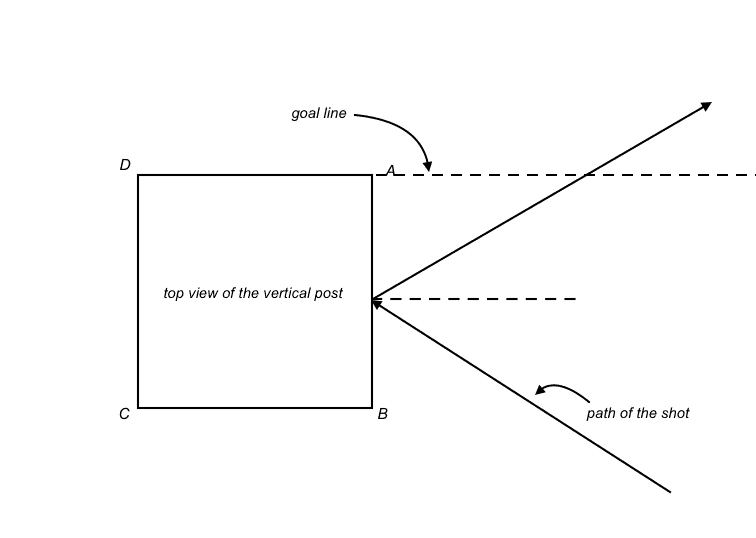Now the interesting case of circular goal post,
In the figure below (top view of the goal post), any shot hitting the sector ADC will never go in, any shot hitting sector AB will always go in and any shot hitting sector BC will go in only when the angle of incidence is greater than the critical value. The critical value of angle of incidence happens when the reflected ray from the sector BC of one post hits the sector AB of the other post.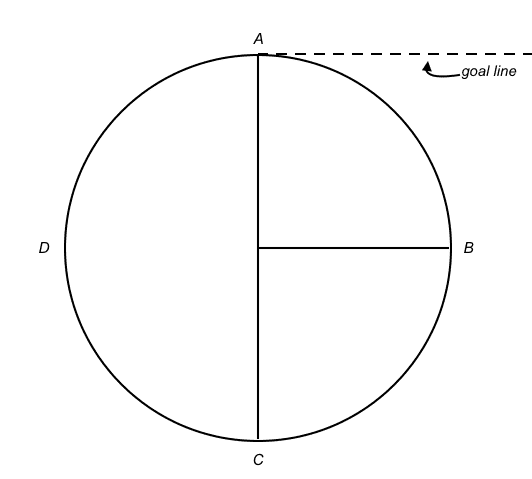Limiting/critical incidence,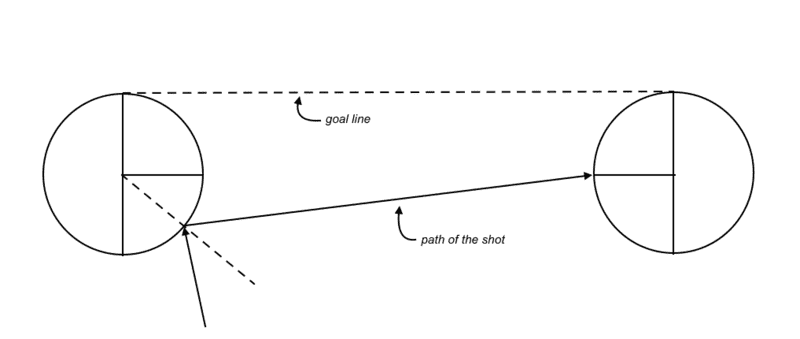So now all we have to do is find the range of all the values of angle of incidence ##\theta## in sector BC for which the shot goes in i.e., [##\theta_{critial}##,##\frac \pi 2##] and then divide that by range of all permit-able values of ##\theta## i.e., [0,##\frac \pi 2##]

The problem is that this range of possible values of ##\theta## changes at every point on sector BC. And even though the length between posts and the radius of the posts are fixed, the value of ##\phi## (shown below) changes from [0,##\frac \pi 2##]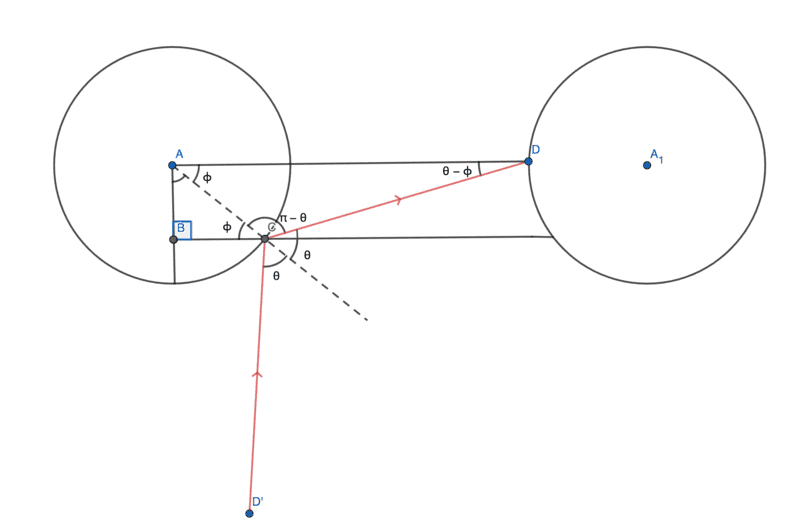Now we can get a relation between ##\phi## & ##\theta## using sine law in ∆ADC,
$${\frac {\sin(\theta-\phi)} {AC}}={\frac {\sin(\pi-\theta)} {AD}}$$
here AC & AD are known (radius & distances between the posts respectively) and are constant but ##\theta## & ##\phi## are both variables

So that is my question, I want to find the critical value of ##\theta## for every value of ##\phi \in [0, \frac \pi 2]## i.e., first I want to individually calculate the probability of a shot going in for a particular value of ##\phi## then I'll add all the probabilities for every value of ##\phi \in [0, \frac \pi 2]##

So somehow I need to find,
$$\sum_{\phi=0}^\frac \pi 2 (\frac \pi 2 - \theta_{critical})$$
but the value of ##\theta## is non-linear and I don't know how to find that summation.

I don't know if this is the right way to do this or if there is any way of doing this?
In the end all I wanted was to find which shape of goal post is better? Square or circular, for most of the shots hitting the post, leading to a goal.
Any help is appreciated :)

Edit: to add a few assumptions I made in this post, the ball is a point mass moving with uniform speed in horizontal plane i.e., there is no vertical component of velocity of the ball, the ball is equally likely to come from any angle, there is no affect of spin on the ball, there is no friction at the point of collision.

The intent of this post was to find out that under similar conditions (i.e., under the assumptions I made) if we compare the square post with the circular one, which post is more likely to give us more goals after striking the post, that's it. I'm not interested in all possible ways of scoring goal via hitting the post, I just want the comparison that which of the two types of posts are better i.e., which of the two will offer us more goals!

Last edited:

Gold Member
Just a minor correction. Soccer does not use a post. Soccer uses a net.

•PeroK
kshitij
Just a minor correction. Soccer does not use a post. Soccer uses a net.
By post I meant goal posts, the vertical and horizontal poles between which the ball is supposed to enter to score a goal

Staff Emeritus
Homework Helper
Gold Member
Homework Statement:: Consider two shapes of goal posts, one with a circular cross-section and the other with square. Which of these posts have a greater probability of a shot hitting the post, go in?
Relevant Equations:: Laws of reflection

First let's talk about the square post,
it is clear that the shot will go in only if the ball hits the side AB of the square shown below, so the probability of a shot going in is simply 13 as sides AB, BC & CD are equivalent and only hitting one of these 3 sides leads to a goal.
There are a lot of things wrong with this reasoning. First of all, the basic assumption is incomplete. Whether the ball goes into the net or not is going to depend on the angle that the ball hits in and therefore on the distribution of balls coming in at each angle. Furthermore, hitting a side is not the only way of hitting a square post, there is also going to be some probability of hitting one of the corners without actually hitting the sides.

Similar arguments are valid for the circular goal posts, there are many unknowns that you'll need to specify distributions for and you'll need to specify how the posts are to be compared.

kshitij
Whether the ball goes into the net or not is going to depend on the angle that the ball hits in and therefore on the distribution of balls coming in at each angle
But in case of square post, why should it depend on the angle that the ball hits it? If the ball hits the side AB (as shown in the first figure) then it should always cross the goal line I cannot see how it won't

hitting a side is not the only way of hitting a square post, there is also going to be some probability of hitting one of the corners without actually hitting the sides.
Yes I ignored the ball hitting the corner because the ball should never go in after hitting the corner the only way for the ball to go in (according to me) is hitting on the side AB (see first figure)

Staff Emeritus
Homework Helper
Gold Member
But in case of square post, why should it depend on the angle that the ball hits it? If the ball hits the side AB (as shown in the first figure) then it should always cross the goal line I cannot see how it won't
You're misunderstanding my point. Depending on the angle, there will be different cross sectional areas to hit each side - and to hit the corner.

Yes I ignored the ball hitting the corner because the ball should never go in after hitting the corner the only way for the ball to go in (according to me) is hitting on the side AB (see first figure)
You can't just ignore it. It is a possibility and will affect the probability. And again, you will need the distribution of incoming balls.
Edit: you could also hit the inner corner ...

kshitij
Depending on the angle, there will be different cross sectional areas to hit each side - and to hit the corner.
I'm sorry but I can't understand what you're saying?

Also I didn't mention a lot of others things in my post like I assumed the ball to be a point mass for simplification and the ball always moves in a straight line without any spin

kshitij
Edit: you could also hit the inner corner ...
Hitting that corner isn't possible in legal play

Staff Emeritus
Homework Helper
Gold Member
Hitting that corner isn't possible in legal play
That's just wrong.

kshitij
That's just wrong
I mean for hitting that corner the ball must come from behind the goal line (which isn't legal play) otherwise for any incidence close to that corner where the ball comes from the front of the goal line, the normal about which the ball will be reflected will be same as for any other point on side AB so that incidence is basically same as hitting the side AB

Thats why I said hitting that corner from legal play isn't possible. I can still be wrong though so feel free to correct me.

Homework Helper
I mean for hitting that corner the ball must come from behind the goal line (which isn't legal play)
There are four corners on a four-sided goal post.

Inside front (facing between the field and the mating goal post)
Inside back (facing between the net and the mating goal post)
Outside front (facing between the field and the side-lines)
Outside back (facing between the side-lines and the out-of-play back field).

I assume that we are speaking here of the inside front corner. A soccer ball with non-negligible size can strike this corner and bounce out. It can also strike this corner and bounce in. Either result can be obtained with a ball arriving from in front of the goal.

On re-reading, I think @Orodruin is talking about the inside back corner of the post. It is indeed possible to strike that corner with a ball hit in from the field of play. [Of course, absent some pretty impressive spin, the result is almost certainly going to be a goal].

Last edited:
Gold Member
I apologize for misunderstanding your intention. I am guessing that you are intentionally ignoring (1) the vertical component of a balls motion, including bounces off the ground, and (2) the spin on the ball. That is, are you restriction that ball paths to straight lines except for their bounces off a vertical post. Is this correct? I am also guessing that you are assuming that a side of the square posts posts is along the goal line, the circular posts are positions to be tangent to and inside the goal line, and that the speed of a ball is irrelevant.

Also, as @Orodruin has said, to make the desired calculation you need to start with some assumption of the probability distribution of the relevant components of the trajectories of the balls which hit a post. One example of a distribution is to assume the ball is kicked from a point on a line at a fixed distance from the goal line and the the probability of a point from which the ball is kicked is uniform along the line. Another possibility is to assume that the probability of the range of angles between the ball's trajectory and the line from which it is kicked is uniform. To make the calculation you want, you need to choose a probability distribution.

kshitij
I am guessing that you are intentionally ignoring (1) the vertical component of a balls motion, including bounces off the ground, and (2) the spin on the ball. That is, are you restriction that ball paths to straight lines except for their bounces off a vertical post. Is this correct? I am also guessing that you are assuming that the square posts are aligned with the imagined lines connecting the front posts and that the speed of a ball is irrelevant.
YES! I forgot to mention about the vertical component sorry.

kshitij
Also, as @Orodruin has said, to make the desired calculation you need to start with some assumption of the probability distribution of the relevant components of the trajectories of the balls which hit a post. One example of a distribution is to assume the ball is kicked from a point on a line at a fixed distance from the goal line and the the probability of a point from which the ball is kicked is uniform along the line. Another possibility is to assume that the probability of the range of angles between the ball's trajectory and the line from which it is kicked is uniform. To make the calculation you want, you need to choose a probability distribution.
Can't we just use the angle with which the ball hits the post to calculate the probability?

Like I'm not interested in the speed of the ball or the distance of the ball from the post, I'm only interested to find the angles of incidence for which the ball will end up behind the goal line assuming there is no spin the ball is a point mass, the collision is elastic, etc.

Let's say for example we found that for angles 60º to 90º the ball will go behind the goal line and the possible values that angle of incidence could take was from 0º to 90º, so can't we simply divide (90-60)÷90 and say that the required probability is 1÷3 in this case? Is saying that wrong?

kshitij
A soccer ball with non-negligible size can strike this corner and bounce out.
Yes but I assumed the soccer ball to be a point mass to avoid complication sorry for not mentioning that in the post (see the edit on the original post)

Last edited:
Staff Emeritus
Homework Helper
Gold Member
I mean for hitting that corner the ball must come from behind the goal line (which isn't legal play) otherwise for any incidence close to that corner where the ball comes from the front of the goal line, the normal about which the ball will be reflected will be same as for any other point on side AB so that incidence is basically same as hitting the side AB
This is incorrect for a ball of non-zero size.

Staff Emeritus
Homework Helper
Gold Member
Yes but I assumed the soccer ball to be a point mass to avoid complication sorry for not mentioning that in the post
That is a pretty bad approximation of the very real situation you asked about. The ball typically has significant radius relative to the size of the post. But there are yet other issues with the question, such as what distribution of incoming balls you are assuming.

kshitij
such as what distribution of incoming balls you are assuming.
What do you mean by this can you please elaborate?

Staff Emeritus
Homework Helper
Gold Member
What do you mean by this can you please elaborate?
The probability of the ball going into the goal will depend on the angle. Therefore, to arrive at any sort of final probability, you will need to specify how likely it is that the ball comes in at each angle.

kshitij
The probability of the ball going into the goal will depend on the angle. Therefore, to arrive at any sort of final probability, you will need to specify how likely it is that the ball comes in at each angle
Oh so that's what you meant, it is equally likely to come from every angle. As I mentioned in the edit of the original post, I'm not interested in exploring all types of possibilities of scoring after rebound.

I want to compare those two styles of post under similar conditions, that's why I made these assumptions.
I'm only interested in finding out which post is better to score goals after rebound under those conditions!

You could argue that the assumptions are far from reality but considering these factors like size of the ball, spin of the ball, probability of the balls coming from each angle, etc, will make things way too complicated (it is already complicated enough for me)

That's why I believe, these assumptions should help us give a good enough idea about which shaped-post is better.

Homework Helper
Gold Member
2022 Award
That's why I believe, these assumptions should help us give a good enough idea about which shaped-post is better.
It hardly matters since the posts these days are officially elliptical!

https://www.fourfourtwo.com/features/weird-and-wonderful-history-goal-posts

The square designs were eventually banned by FIFA in 1987, and the round version has also been gradually replaced by an elliptical shape created by the Standard Goals Company in Nottingham.

•rsk
Homework Helper
Gold Member
Yes but I assumed the soccer ball to be a point mass to avoid complication sorry for not mentioning that in the post (see the edit on the original post)
Which is wider: the ball or the post? So if you want to simplify the problem by assuming one of their widths is negligible, which should it be? How does this affect the answer to your question?

•hutchphd
Homework Helper
Gold Member
Just a minor correction. Soccer does not use a post. Soccer uses a net.
No, the posts and crossbar are specified in the rules; the net is optional.

•hutchphd
Homework Helper
Gold Member
It hardly matters since the posts these days are officially elliptical!

https://www.fourfourtwo.com/features/weird-and-wonderful-history-goal-posts

The square designs were eventually banned by FIFA in 1987, and the round version has also been gradually replaced by an elliptical shape created by the Standard Goals Company in Nottingham.
If that is true then they must have subsequently been unbanned: from the 2020/21 IFAB Laws of the Game:

10 Goals... [The goalposts and crossbar] must be square, rectangular, round, elliptical or a combination of these shapes and must not be dangerous...

•hutchphd
Homework Helper
Gold Member
2022 Award
Which is wider: the ball or the post? So if you want to simplify the problem by assuming one of their widths is negligible, which should it be? How does this affect the answer to your question?
To elaborate on that, in practice, the ball is wider than the post. If we consider the ball coming equally likely from all directions and equally likely on any lateral displacement (so long as it hits the post) then I suggest it is more likely to hit a corner of the post than a face. And even though the ball is coming from within the bounds of the pitch, any of the four corners of the post can be struck - but from any given direction, at most three corners are possibilities.
Unless the ball comes from the very end of the pitch, there is the possibility of striking a corner of a post and yet continuing into the goal.

Homework Helper
Gold Member
To elaborate on that, in practice, the ball is wider than the post.
Yes, and when you take into account deformation of the ball* in contact then to talk about hitting a corner or a face at all is a gross oversimplification. Either you take these factors and more into account (friction, the ball's moment of inertia, elastic coefficient...), at which stage I would suggest that any physical model is going to be less reliable than experiment, or you don't and you conclude that the shape does not make any predictable difference.

* see for example https://www.pond5.com/stock-footage...ts-bar-and-bounces-back-slow-motion-beautiful

kshitij
you conclude that the shape does not make any predictable difference.
Well, the whole point of the this thread was finding out this particular question, because I've heard people say that a lot more came out than went in with the square posts and that's why they were replaced by round ones.

I wondered whether this could be simply proved using some assumptions and geometry and therefore decided to post this thread.

Homework Helper
Gold Member
2022 Award
Well, the whole point of the this thread was finding out this particular question, because I've heard people say that a lot more came out than went in with the square posts and that's why they were replaced by round ones.

I wondered whether this could be simply proved using some assumptions and geometry and therefore decided to post this thread.
Since the ball is wider than the post I considered a post of negligible width. Averaging over all directions (i.e. ##\pm \pi/2##) and all lateral displacements (i.e.##\pm R##, the radius of the ball), I get a probability of going in of ##\frac 12-\frac{\sqrt 2}{\pi}\approx \frac 1{20}##, regardless of post shape.
So to find which has the higher probability, it seems reasonable to go to the other extreme and treat the ball as a point.
But now the question is which dimension of a square post should we use in comparing with a round one? Should it have the same minimum width, the same maximum (diagonal) width, or the same cross-sectional area?

kshitij
I get a probability of going in of 1/2−√2/π≈1/20, regardless of post shape
How did you get that?
But now the question is which dimension of a square post should we use in comparing with a round one? Should it have the same minimum width, the same maximum (diagonal) width, or the same cross-sectional area?
Well in my post I used that the diameter of the circular area should be equal to the side length of the square post. This is according to the official laws of the game. (see page 21 of the pdf)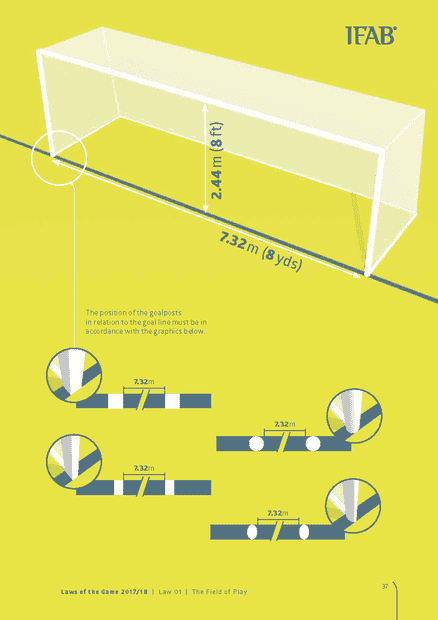The boundaries of the goal post must lie within the width of goal line which is 12cm.
Thus, the diameter of the circular post = edge length of the square post = 12cm.

Initially I wanted to compare even the rectangular post & the elliptical post as well, but their dimensions are not known so I ignored them.

•PeroK
Homework Helper
Gold Member
2022 Award
It seems that a shot coming in at an angle only needs to hit the inside of a square post to go it; whereas, the ball may hit the inside of a circular or elliptical post and stay out. For an acute enough angle it becomes impossible to go in off the curved post.

•kshitij
kshitij
It seems that a shot coming in at an angle only needs to hit the inside of a square post to go it; whereas, the ball may hit the inside of a circular or elliptical post and stay out. For an acute enough angle it becomes impossible to go in off the curved post.
I agree completely with you here, and that acute angle is what I intended to find in my post but couldn't because the math got too complicated for me.

Homework Helper
Gold Member
2022 Award
I agree completely with you here, and that acute angle is what I intended to find in my post but couldn't because the math got too complicated for me.
I think it is complicated. It we only consider a square post and a ball shot directly at the goal (perpendicular to the goal-line), then the effective size of the goal depends on the size of the ball. The point-sized ball would go in as long as it avoids the post. But, a finite ball may bounce out unless its centre is sufficiently within the post. For a round post, you'll need the diameter of the ball and the post.

You could, perhaps, consider that case first. Then, try to generalise to a shot coming in at an angle.

kshitij
I think it is complicated. It we only consider a square post and a ball shot directly at the goal (perpendicular to the goal-line), then the effective size of the goal depends on the size of the ball. The point-sized ball would go in as long as it avoids the post. But, a finite ball may bounce out unless its centre is sufficiently within the post. For a round post, you'll need the diameter of the ball and the post.

You could, perhaps, consider that case first. Then, try to generalise to a shot coming in at an angle.
I only need a rough idea about which post is better.

If say somehow we could conclude that the round post is better than the square one for a point sized ball, then I would be happy to say that it should also be better for finite sized ball even though this might not be true but unless one could prove that the square post is better in the case of finite sized ball why should I believe it? I got my rough idea, I'm happy.

Homework Helper
Gold Member
2022 Award
I only need a rough idea about which post is better.

If say somehow we could conclude that the round post is better than the square one for a point sized ball, then I would be happy to say that it should also be better for finite sized ball even though this might not be true but unless one could prove that the square post is better in the case of finite sized ball why should I believe it? I got my rough idea, I'm happy.
My guess is that it depends on the angle of the shot: round may be better than square posts for a direct shot and square better than round for a shot from an acute angle.

One proviso is the complexity of a collision between a ball and the corner of a square post - and the friction between the ball and post in general. It won't be an elastic collision.

kshitij
My guess is that it depends on the angle of the shot: round may be better than square posts for a direct shot and square better than round for a shot from an acute angle.
What do you mean by direct shot? a shot perpendicular to the goal line? If that's the case then the ball shouldn't go in for either square or round post, as it is a point sized ball.

So, if we have to compare them then it is obviously comparing the angled shots.